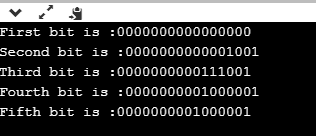# How to use bitset in C++

In this post, we will learn what exactly is a bitset in C++? And how we can harness the power of it and use it to make great things. So what exactly is bitset?

A bitset is a large dataset or array of GUI type that stores data as only boolean values (either 0 or 1). You can perform different operations on this array. The space occupied is very less as compared to the integer or other arrays.

We can easily see through the help of this program that how the data that we insert goes on in the brain of the computer or the processor. The processor processes such data after converting each and everything into its binary equivalent.

## Algorithm to demonstrate bitset in C++

1. Declare bitset<16> b1=9
2. Print b1
3. Declare bitset<16> b2=’A’
4. Print b2
5. Declare bitset<16> b3=65
6. Print b3
7. Declare bitset<16> b4=1010010101
8. Print b4
9. End

## Program: use bitset in C++

```// Example program
#include <iostream>
#include <string>
#include<bitset>
using namespace std;
int main()
{
bitset<16> b1;
bitset<16> b2=9;
bitset<16> b3='9';
bitset<16> b4='A';
bitset<16> b5=65;

cout<<"First bit is :"<<b1<<endl;           //This prints the initial value stored in an empty bitset
cout<<"Second bit is :"<<b2<<endl;          //This shows the bit value of the number 9 translated from hexadecimal value
cout<<"Third bit is :"<<b3<<endl;           //This translated from the character '9' (notice these values are different from the previous)
cout<<"Fourth bit is :"<<b4<<endl;          //This is the bit value of ASCII letter A
cout<<"Fifth bit is :"<<b5<<endl;             //Notice that the ASCII value of A produces the same result

}
```

#### The output of the following program:This is a very interesting and fun program. You can experiment with different bit values of the various data that you insert be it integer, character or string.